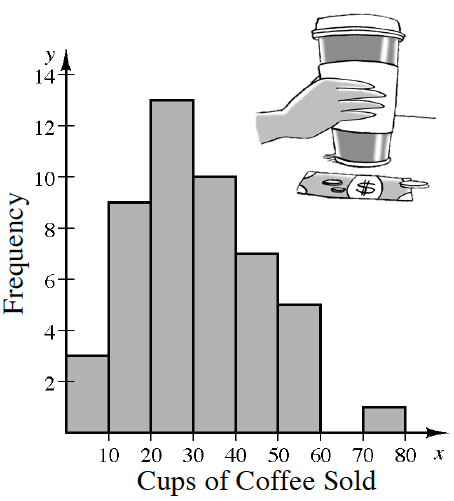### Home > INT3 > Chapter 1 > Lesson 1.2.3 > Problem1-103

1-103.

Katelyn owns a $24$-hour coffee shop in front of a downtown office building. She recorded the number of cups of coffee sold each hour during a $48$-hour time period in the table below.

Katelyn sorted the data and made the histogram below.

 Cups of Coffee Sold $1$ $22$ $37$ $5$ $22$ $38$ $7$ $23$ $38$ $12$ $24$ $40$ $12$ $25$ $41$ $12$ $26$ $41$ $12$ $26$ $42$ $12$ $29$ $47$ $14$ $29$ $47$ $16$ $30$ $49$ $17$ $30$ $51$ $19$ $31$ $52$ $20$ $32$ $55$ $21$ $33$ $55$ $21$ $35$ $59$ $22$ $36$ $76$ checksum $1444$1. Using the sorted data from the table and the histogram, and without using your calculator, what is the five-number summary (minimum, first quartile, median, third quartile, maximum)?

For quartiles:
Look between the $19$ and the $20$.
Look between the $29$s.
Look between the $40$ and the $41$.

Minimum: $1$
Maximum: $76$

2. Describe the distribution of coffee sales per hour

What would be the typical number of cups sold in an hour?
Does the distribution have skew? Is there an outlier?
What is the IQR? What would it represent?